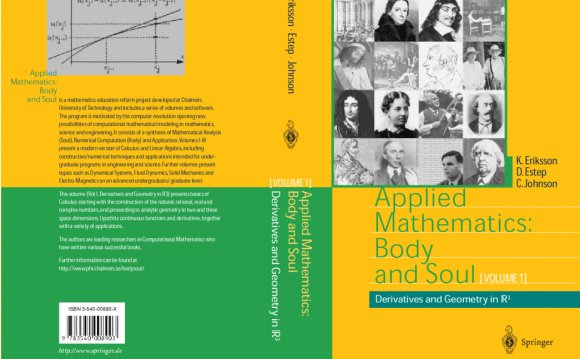# Applied Mathematics body and soul

November 16, 2023Preliminary version
Publisher page

#### In Brief

In this book we address mathematical modeling of turbulent fluid flow, and its many mysteries that have haunted scientist over the centuries; such as the dAlembert mystery of drag in an inviscid flow, the Sommerfeld mystery of transition to turbulence in shear flow, and the Loschmidt mystery of violation of the 2nd law of thermodynamics. We approach these mysteries using a synthesis of computational and analytical mathematics, and in the book we offer new solutions to these mysteries that we believe are more to the point than traditional ones. In many ways the material in this book goes against what is traditionally found in most text books of mathematical fluid dynamics.
This book has its own dedicated homepage where we make movies available from the many computations in the book, which we hope will be entertaining as well as lead to new insights. Since our approach is new, and may also be considered controversal by some, at the homepage of the book we open for a free debate on these very important questions that are fundamental for both mathematics and fluid dynamics.

&nbsp

### Dreams of Calculus - Perspectives on Mathematics Education

The new book Dreams of Calculus - Perspectives on Mathematics Education is now available in postscript and pdf format.
Publisher page

In this book we argue that the development of the computer and the rapid increase in available computational power constitutes a paradigm shift in mathematics, with dramatic new possibilities of computational mathematical modeling in our society. We further argue that this paradigm shift must lead to a corresponding paradigm shift in mathematics education on all levels.

Roughly speaking, the book contains a synthesis of calculus and linear algebra including computational methods and a variety of applications. Emphasis is put on constructive/computational methods with the double aim of making the mathematics both understandable and useful.

Our ambition is to introduce the student early to both (from the perspective of traditional education) advanced mathematical concepts (such as Lipschitz continuity, Cauchy sequence, contraction mapping, initial-value problem for systems of differential equations), and advanced applications such as Lagrangian mechanics, n-body systems, population models, elasticity and electrical circuits, with an approach based on constructive/computational methods. The idea is thus that making the student comfortable with both advanced mathematical concepts and modern computational techniques, will open a wealth of possibilities of applying mathematics to problems of real interest.

This is in contrast to the traditional education where the emphasis is usually put on a set of analytical techniques within a conceptual framework of more limited scope. For example: we lead already in the second quarter the student to write (in Matlab) his/her own solver for general systems of ordinary differential equations based on mathematically sound principles (high conceptual and computational level), while traditional education at the same time often focuses on a training the student to master a bag of tricks for symbolic integration. We also teach the student some tricks to that purpose, but our over-all goal is different.

#### Upcoming

Computational Thermodynamics: (Vol. 5)
Dynamical Systems: (Vol. 6)
Solid Mechanics: (Vol. 7)
Electromagnitics: (Vol. 8)
[to appear 2006/7]

This book presents a unified approach to computational mathematical modelling based on differential equations combining aspects of mathematics, computation and application. The backbone of the book is a general methodology for the numerical solution of differential equations based on Galerkin's method using piecewise polynomial approximation.

The book is a substantial revision of the successful text Numerical Solution of Partial Differential Equations by the Finite Element Method by C. Johnson. It begins with a constructive proof of the Fundamental Theorem of Calculus that illustrates the close connection between integration and numerical quadrature and introduces basic issues in the numerical solution of differential equations including piecewise polynomial approximation and adaptive error control. After preparatory material on linear algebra and polynomial approximation, the computational methodology is developed starting with model problems taking the form of scalar linear ordinary differential equations, then proceeding through systems of linear ordinary differential equations to linear partial differential equations including the Poisson equation, the heat equation, the wave equation and convection-diffusion-absorption equations. The book includes background material on the derivation of the differential equations as mathematical models of physical phenomena and mathematical results on properties of the solutions of differential equations. The theme of error estimation and adaptive error control is developed consistently.

This text is suitable for courses in mathematics, science, and engineering ranging from calculus, linear algebra, differential equations to specialized courses on computational methods for differential equations. The book is written in an accessible style and includes all of the necessary background material from calculus, linear algebra, numerical analysis, mechanics, and physics. As instructional aids, problems are interspersed throughout the text and the book is complemented by the freely available software Femlab that can serve as a laboratory for the student.

Source: www.bodysoulmath.org
##### INTERESTING VIDEOSound Therapy - Healing your Mind, Body & Soul6 MLK Quotes Applied to Female Body ImageBenny Goodman and Teddy Wilson plays Body and Soul Live in1948